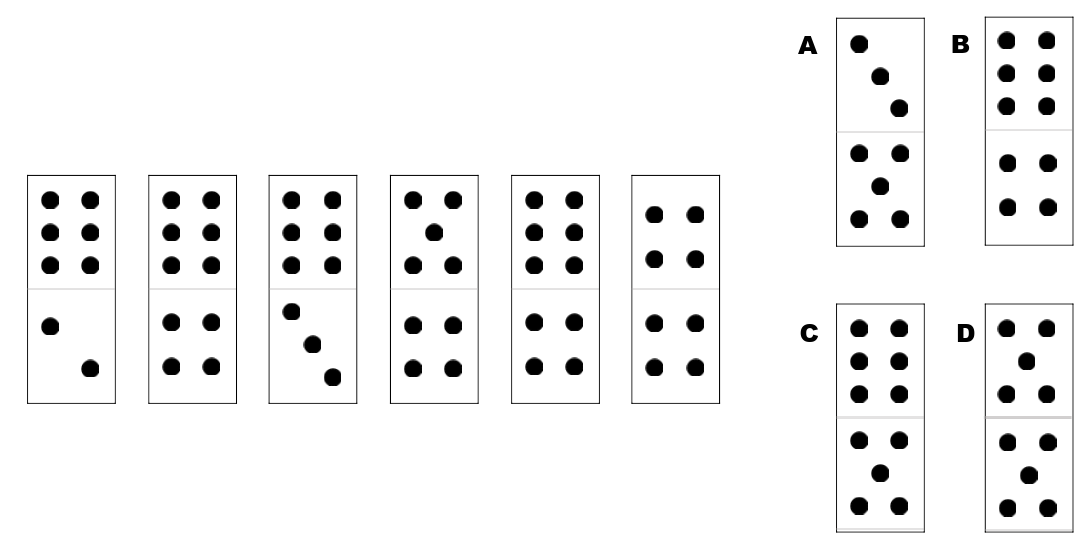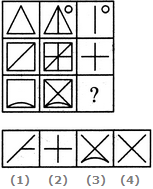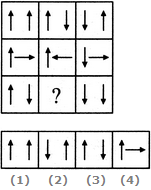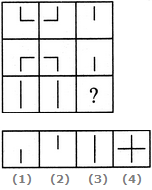> > Odd Figures

# Odd Figures

The reasoning that is asked in competitive exams has many forms. Today we will emphasize on one of the topics covered in abstract reasoning which is odd figures. Abstract reasoning is used to test a candidate’s intellect and the general ability to work out the new concepts. We shall learn about odd figure in this article. Let us do it right now.

### Suggested VideosColoured Cube ISequence of Occurence of Events in a ProcessBlood Relations Introduction## Questions Expected in Odd Figures Test

Odd figures test are more common in the job related competitive exams because it tests the intellectual potential of a candidate. There are also useful for jobs because it gives the employer a chance to see the person’s problem-solving skill because a candidate will be required to work under the pressure with some complex data and perform the required tasks. It can be for any position as it is a good parameter to test the general intelligence, and gives a chance to look into your ability to serve the spatial relationships and work out your way. To be able to complete these type of tests you need to use your lateral thinking and be creative. You also need to find the relationship between figures and shapes, identify the pattern and quickly answer the question.

### Browse more Topics under Classification

Odd figures are designed to be challenging and to differentiate the candidates and identify the best performance they are capable of. These questions can be time-consuming. And that is why it is important that you have enough practice so that you can increase your speed and solve these questions quickly. This means that you need to solve more and more questions of this type and develop a rule so that even though the complexity increases your effort and time does not.Odd figures

Now we will help you with some solved problems which can help you understand the theme of the questions. We have divided the questions based on their type and method required to solve.

### Identification of the missing squaresIn this type of questions, you are required to look at the patterns and formation that are prepared in the squares. The question asks you to find the missing square. Thus it is important that you understand the relationship between the squares in the question.

There are three objects in the question. You can pick one object and identify its pattern in the squares and then you can predict it’s position in the missing square. This can be your answer. You can do the same process for other two squares. This will ensure that your answer is correct.

Here in this question, the black square moves from left to right in each row. Thus you have established its position in the missing square. Now you can look at the movement of the circle in all the squares. It is in the clockwise direction from left to right. And finally, the position of the error in first and square in every is a reflection of each other. Thus we have determined the position of all the objects in the missing square. Hence the correct answer is C.

## Identifying the sequenceIn this type of question, you are required to identify the pattern and determine what next will be followed. Here in the figure, you can see there are two patterns. In the first pattern, the first, third, and fifth figure have six in the upper box common and there is a subsequent increase of one dot in each of the lower boxes. Thus the seventh box will have five dots in the lower box. Therefore, for the second pattern, the remaining boxes i.e. second, fourth, and sixth have the same number of dots in the lower boxes, but there is a subsequent decrease of the dot in each of the above boxes. Hence, the correct answer here is C.

#### A is to B, as C is to DIn these types of questions, you need to find the relationship between the given data. Just like in this question, first, you need to determine how are 1 and 2 related. Then apply the same pattern to the 3rd and 4th figure. When you look at the figure with more concentration you will notice that in 1 and 2 the number of codes is increasing. Figure 1 has 7 sides while figure 2 has 8 sides. Thus figure 4 will have one side greater than the 3rd figure i.e. 11 sides.

The second indication here is the direction of the arrow. In figure 1 and 2, the arrow is completely opposite to each other. Same will be followed for 3rd and 4th figure. Thus the correct answer here is A.

## Practice Questions

Q. In the given question find out the missing figure which will follow the given figures.1. 1                    2. 2

3. 3                   4. 4

Q. Based on the given arrows in the squares find out the missing figure.1. 1                    2. 2

3. 3                   4. 4

Q. Which figure out if the given four figures will complete this matrix in the question?1. 1                   2. 2

3. 3                  4. 4

Share with friends

## Customize your course in 30 seconds

##### Which class are you in?
5th
6th
7th
8th
9th
10th
11th
12th
Get ready for all-new Live Classes!
Now learn Live with India's best teachers. Join courses with the best schedule and enjoy fun and interactive classes.Ashhar Firdausi
IIT Roorkee
BiologyDr. Nazma Shaik
VTU
ChemistryGaurav Tiwari
APJAKTU
Physics
Get Started

## Browse

##### ClassificationSubscribe
Notify of

## Question Mark?

Have a doubt at 3 am? Our experts are available 24x7. Connect with a tutor instantly and get your concepts cleared in less than 3 steps.